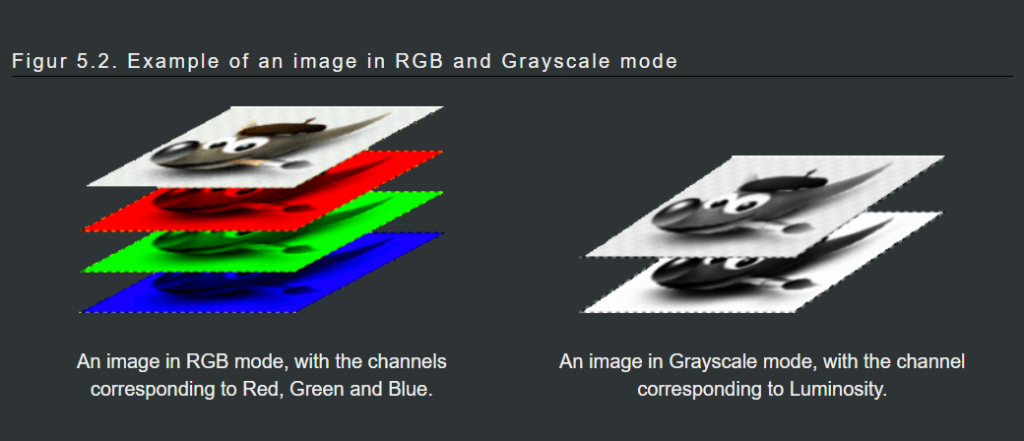# Load Different layers of images

A grayscale image and an RGB image is the number of “color channels”: a grayscale image has one.

An RGB image has three. An RGB image can be thought of as three superimposed grayscale images, one colored red, one green, and one blue.Lets start to play with an image, take a image and abstract the information of the image, we are going to take an image of three colors like below:```#Load the important libraries.

import numpy as np
from PIL import Image

img = Image.open("cv.png")
nparray=np.array(img)

#Check the shape of image

nparray.shape

#(739, 600, 4)

#Abstract the information from each layer, as we have seen that this image is having three layers.

r=nparray[:,:,0]
g=nparray[:,:,1]
b=nparray[:,:,2]
o=nparray[:,:,3]
#Take a zeros matrix of same shape
z1=np.zeros_like(r)
z2=z1+255
z3=z1+255
#Use Dstack-depth stack
imgData=np.dstack([z1,r,g])
imgnew=Image.fromarray(imgData,'RGB')
imgnew.show()```

So the output is like: